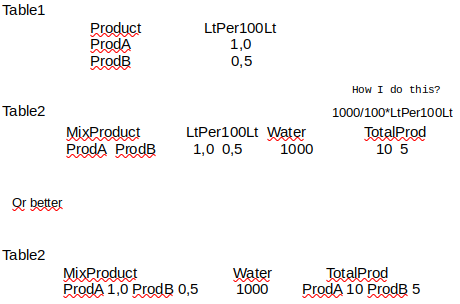# Sum Reference list

First of all congratulations for this great project.Thanks

Hi there and welcome to the Community!

https://public.getgrist.com/v4vj2PDZS4jf/Community-665/p/1/m/fork

Table 1 lists the products and Lt per 100 Lt

Table 2 shows the Mix Product (Reference List to the Product column in Table 1).

Lt per 100 Lt is a formula column that pulls data from the Lt per 100 Lt column in Table 1. Because the Mix Product column is a reference list column, I can only pull in the product name. This is why the Lt per 100Lt column is still in in table 2. This column is not used in the calculation of Total Prod so you could remove this column if you’d like.

Water = 1000.

Total Prod column is a formula column. I was able to get this in the format of Prod A 10 Prod B 5.

Total Prod Formula:

``````mix_list_str = \$Mix_Product.Lt_per_100_Lt
mix_list_float = [float(i) for i in mix_list_str]
x = [Lt * \$Water/100 for Lt in mix_list_float]
l = \$Mix_Product.Product
' '.join('{} {}'.format(first, second) for first, second in zip(l, x))
``````

Let me know if you have any questions!

Thank you for your help, have a nice day.
Regards, A.S.

1 Like

Hi,
another question on this same topic, how can I put ROUND(x, 2)
Thanks

I’ve updated the example document with a new column that shows the use of ROUND in the Total Prod answer.

https://public.getgrist.com/v4vj2PDZS4jf/Community-665

The formula in the Rounded Value column is:

``````mix_list_str = \$Mix_Product.Lt_per_100_Lt
mix_list_float = [float(i) for i in mix_list_str]
x = [Lt * \$Water/100 for Lt in mix_list_float]
round_x = [ROUND(num, 2) for num in x]
l = \$Mix_Product.Product
' '.join('{} {}'.format(first, second) for first, second in zip(l, round_x))
``````

The new part of this formula is `round_x = [ROUND(num, 2) for num in x]`. Because x is a list, we have to round each value (num) in the list.

Then, the join formula must be updated to include the rounded values from round_x instead of x.
’ ‘.join(’{} {}’.format(first, second) for first, second in zip(l, round_x))

In the screenshot below, you’ll see the original ‘Total Prod’ column and the new Rounded Value column. I changed the values for Lt per 100 Lt so you could see the difference. When the value is an integer like it was previously (10.0 and 5.0), it will only show a single decimal even if you have 2, 3, etc listed as the round-to value.

Hi,
another question on this same topic, how can I sum(TABLE2.lookupRecords(Product=\$id).Total Prod)
Thanks

Hello again!

I have updated our example, linked below.

https://public.getgrist.com/v4vj2PDZS4jf/Community-665/m/fork

I added a new column in Table1 that sums the Total Prod values from Table2 for each product.

The formula used here is:

``````water_values = Table2.lookupRecords(Mix_Product=(CONTAINS(\$id))).Water
water_float = [float(i) for i in water_values]
Lt = float(\$Lt_per_100_Lt)
x = [Lt * water/100 for water in water_float]
return SUM(x)
``````

First line of our formula finds all records in Table2 that contain the ID of the current row in Table 1. We assign this list of records to the variable water_values. In line two, we convert each item in the list to a float value.

Next , we convert the value in the LT per 100 Lt column to a float.

Last, we use the values found above in the same formula that we used in Total Prod column of table2. It’s a much simpler formula to find the values then plug them into the same formula rather than trying to pull the value directly from the Total Prod column.

Last, we find a sum of all of the values.

Let me know if you have any questions!

Hi, thank, have a nice day.